# Plan VI Arabic (& others) Phase

Sam Habiel, Pharm.D./ OSEHRA/ 6 Aug 2019

This is the last blog post in the Plan-VI project. We describe how to apply the work of the last year to new languages. We also take the opportunity as well to show VistA CPRS with a few of these languages. We have previously shown Korean and German. In this post, we will show:

• French
• Italian
• Russian
• Arabic

# Unfinished Business

In the previous blog post (here, or mirrored here), I mentioned that I would leave the exercise of uppercasing/lowercasing non-English languages as an exercise to the reader. However, in preparing a demo for Russian, I needed the code in order for Russian to uppercase properly.

So here it is. You need to add code to the Language file (#.85) and modify XLFSTR. For the language file portion, the code differs between Caché and GT.M/ YottaDB. The code is as follows that needs to be added to the Language file:

Field Number Field Name Caché Code GT.M/YDB Code
10.4 Uppercase Conversion S Y=\$ZCVT(Y,"U") S Y=\$ZCO(Y,"U")
10.5 Lowercase Conversion S Y=\$ZCVT(Y,"L") S Y=\$ZCO(Y,"L")

And here is the modified XLFSTR (now committed to the plan-vi repo):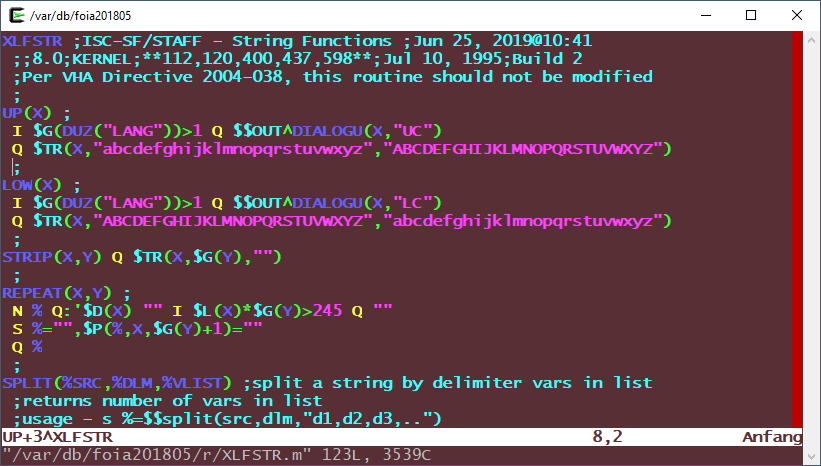XLFSTR with Case Conversions

The added lines are:

``````UP+1: I \$G(DUZ("LANG"))>1 Q \$\$OUT^DIALOGU(X,"UC")
LOW+1: I \$G(DUZ("LANG"))>1 Q \$\$OUT^DIALOGU(X,"LC")``````

# Steps for Implementing CPRS in your own Language

1. Copy CPRSChart.lng file, appending a suffix before .lng for the two letter language name. E.g. CPRSChart.ar.lng for Arabic.
2. Translate the strings after the = sign starting at line 9. For demonstration purposes, I used a routine I wrote (UDEP6TRA) to do machine translations of the file.
3. Create Language file entries for DI, DD, FMTE, LC, and UC for the language. More on that in the next section.
4. Set language in VistA in the DEFAULT LANGUAGE (#207) field in the KERNEL SYSTEM PARAMETERS file (#8989.3); or alternately you can set it at the user level in the LANGUAGE (#200) field in the NEW PERSON (#200) file.

# Language file (#.85) Entries

For the language that you plan to use, you need to set-up the following fields in the language file, as appropriate to your language:

Field Name Field Number Short Name
Date/Time Format 10.2 DD
Date/Time Format (FMTE) 10.21 FMTE
Date Input 20.2 DI
Uppercase Conversion 10.4 UC
Lowercase Conversion 10.5 LC

If your language does not have case, there is no need for you to fill UC and LC. DI will tend to be always the same for most languages (the only exception is that if you already use the US date format in your country; if that case, just leave it blank). And you always put the same code in FMTE as DD. Practically then, you only need to modify DD/FMTE to for each language.

Let's discuss what you need to put in:

UC & LC: discussed above.

DI: `S:\$G(%DT)'["I" %DT=\$G(%DT)_"I" G CONT^%DT`

DD & FMTE: These differ by date output format for language. Here are the languages we put into VistA and their FMTE:

Language Date Format DD & FMTE
German dd.mm.yyyy `S:Y Y=\$S(\$E(Y,6,7):\$E(Y,6,7)_".",1:"")_\$S(\$E(Y,4,5):\$E(Y,4,5)_".",1:"")_(\$E(Y,1,3)+1700)_\$P("@"_\$E(Y_0,9,10)_":"_\$E(Y_"000",11,12)_\$S(\$E(Y,13,14):":"_\$E(Y_0,13,14),1:""),"^",Y[".")`
French dd/mm/yyyy `S:Y Y=\$S(\$E(Y,6,7):\$E(Y,6,7)_"/",1:"")_\$S(\$E(Y,4,5):\$E(Y,4,5)_"/",1:"")_(\$E(Y,1,3)+1700)_\$P("@"_\$E(Y_0,9,10)_":"_\$E(Y_"000",11,12)_\$S(\$E(Y,13,14):":"_\$E(Y_0,13,14),1:""),"^",Y[".")`
Italian dd/mm/yyyy `S:Y Y=\$S(\$E(Y,6,7):\$E(Y,6,7)_"/",1:"")_\$S(\$E(Y,4,5):\$E(Y,4,5)_"/",1:"")_(\$E(Y,1,3)+1700)_\$P("@"_\$E(Y_0,9,10)_":"_\$E(Y_"000",11,12)_\$S(\$E(Y,13,14):":"_\$E(Y_0,13,14),1:""),"^",Y[".")`
Russian dd.mm.yyyy `S:Y Y=\$S(\$E(Y,6,7):\$E(Y,6,7)_".",1:"")_\$S(\$E(Y,4,5):\$E(Y,4,5)_".",1:"")_(\$E(Y,1,3)+1700)_\$P("@"_\$E(Y_0,9,10)_":"_\$E(Y_"000",11,12)_\$S(\$E(Y,13,14):":"_\$E(Y_0,13,14),1:""),"^",Y[".")`
Arabic dd/mm/yyyy `S:Y Y=\$S(\$E(Y,6,7):\$E(Y,6,7)_"/",1:"")_\$S(\$E(Y,4,5):\$E(Y,4,5)_"/",1:"")_(\$E(Y,1,3)+1700)_\$P("@"_\$E(Y_0,9,10)_":"_\$E(Y_"000",11,12)_\$S(\$E(Y,13,14):":"_\$E(Y_0,13,14),1:""),"^",Y[".")`
Korean yyyy/mm/dd `S Y=\$\$FMTE^UKOUTL(Y,\$G(%F))`

# Patient Registration

I did nothing special here. I registered a few patients with non-Engilsh names for testing: SCHÄFER,MARTA (German), ДМИТРИЕВНА,АННА (Cyrillic), and حليم,أبتسام (Arabic). Our changes from the Korean phase of the project to the DPTLK* and XLFNAME* routines that handle name entry needed no further improvements.

# Screenshots

Here are some screenshots I have taken with various languages.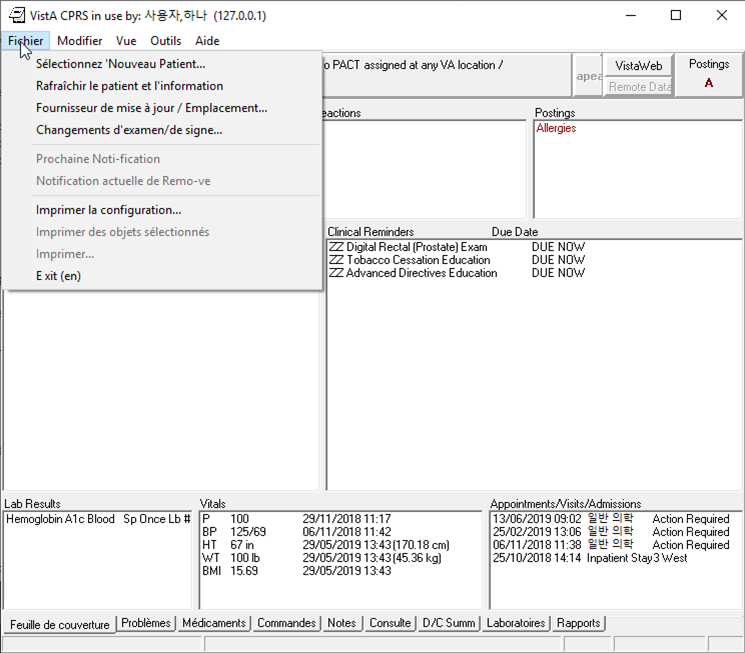French Cover Sheet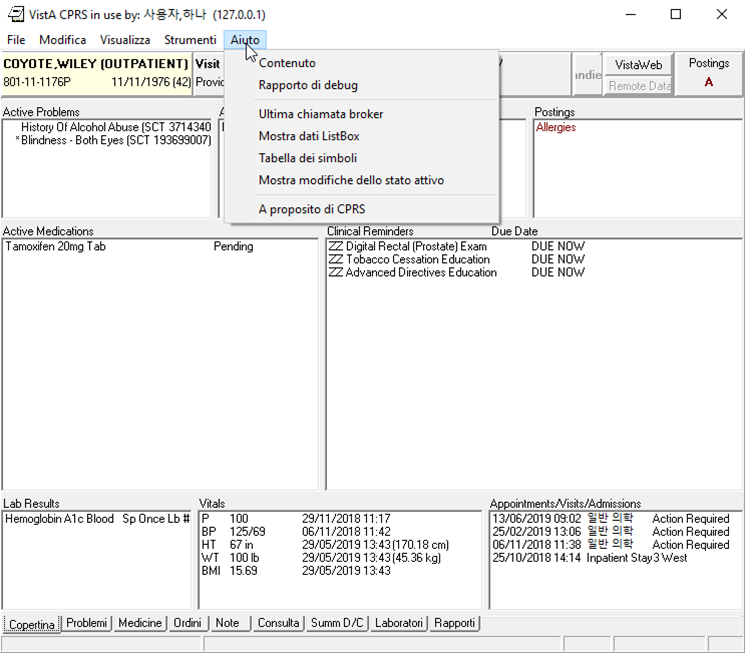Italian Cover Sheet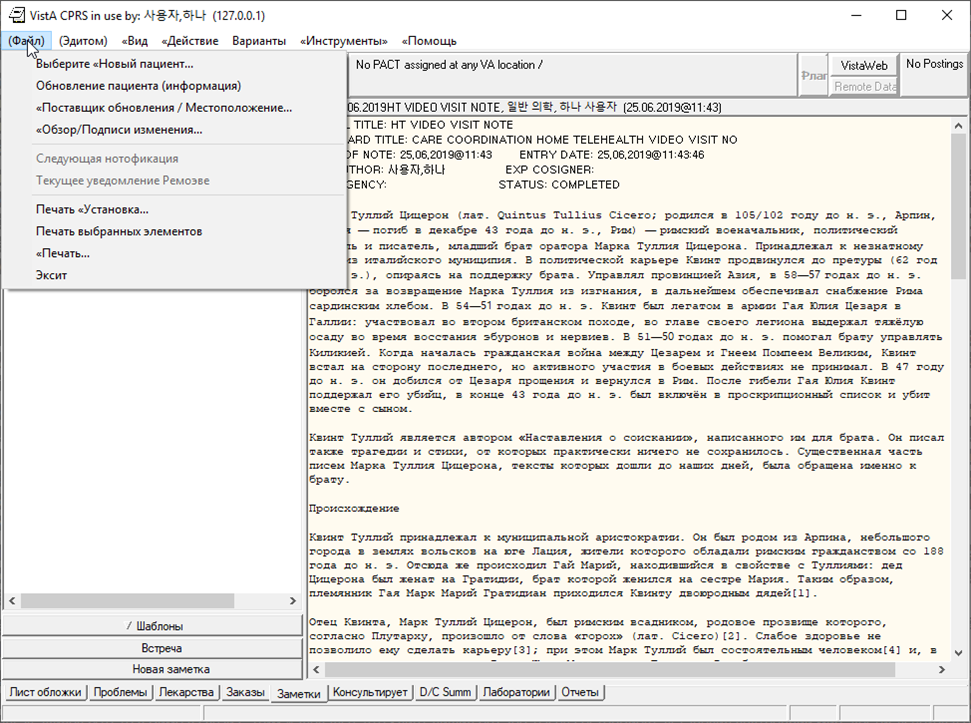Russian Notes Tab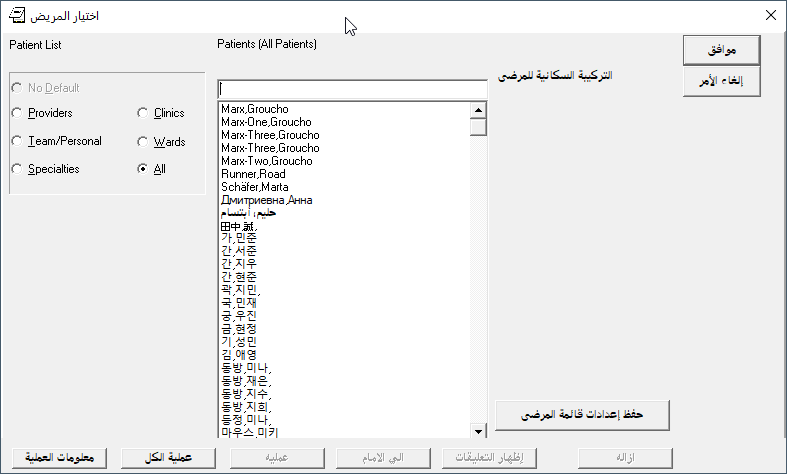Patient Selection show patients in Multiple Languages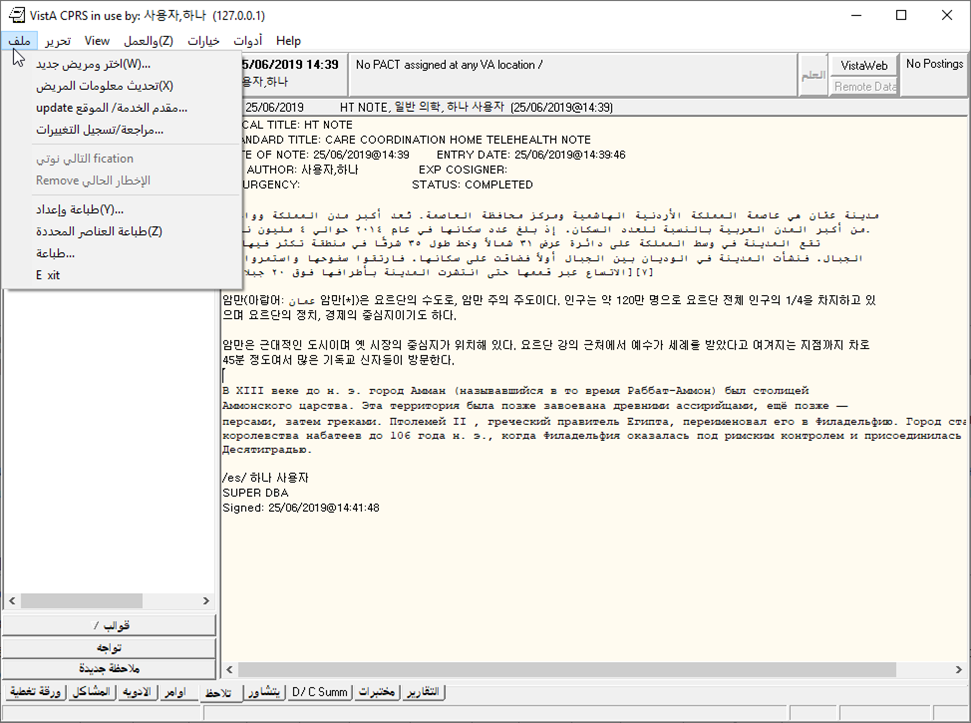Arabic Notes Tab with Note containing Mutiple Languages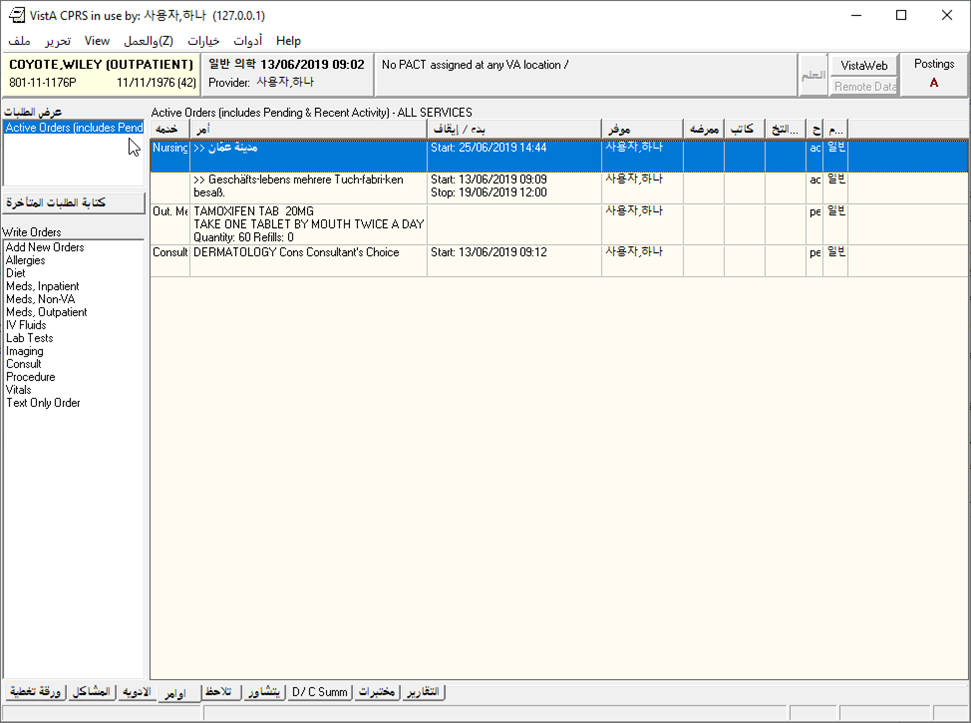Arabic Orders Tab

Side note: I had an unexpected problem with Russian, seen in this screenshot: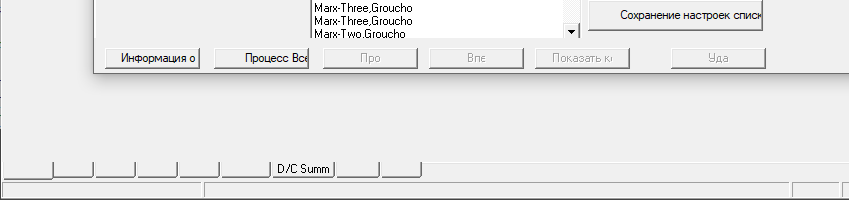Blank labels on Tabs when the text on the tabs is Russian.

This turned out to be a previously reported problem with the font "MS Sans Serif". Apparently it is not a true type font; surprisingly, changing the font of the bottom tabs to the similarly named "Microsoft Sans Serif" fixes the problem.